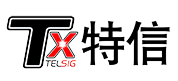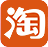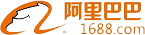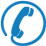﻿ The gain of the signal amplifier? What does dB mean? Do you know? - 【深圳特信电子】
Hello, welcome to visit Shenzhen Texin Electronics Co., Ltd. official website!Focused on the development of cell phone signal amplifiers more than10 yearsNational Free Hotline：
0755-83247232

• News

新闻资讯

Address：No. 228 Xifu Road, Fucheng Town, Pinghu Street, Longgang District, Shenzhen, China
Tel：0755-83247232
Phone：13537825155Position：Home  > News > Common problem >

### The gain of the signal amplifier? What does dB mean? Do you know?

2018-07-05 15:41:19

The gain of the interphone signal amplifier is the multiplier of the radio frequency power of the interphone signal amplifier. Because of the two-way amplifier, there is a uplink gain and a downlink gain, which is basically the same in the normal case. Radio frequency power magnification is expressed in dB, 10dB is 10 times, 20dB is 100 times, 30dB is 1000 times... .., 100dB represents 10000000000=100 billion times

The DB number represents the relative ratio of the signal intensity of the input and output ports of the device (or system).

For example, +10db indicates that the output of the device magnifies the input signal by 10 times.

-10Db indicates that the output of the device has reduced the input signal by 10 times.

Today we will talk to Wang Gong of the Huaan Interfax company to talk about the RF power amplification times of the LD series digital intercom.

1, DBM and dBuV

Both DBM and dBuV are the values of the absolute value of power and level. The formula is: dbm=10lgP (power value /1mw), dbuv=10lgP (level /1uV). Conversion is dbm=dbuv-107 (50 Europe) and dbm=dbuv-108.8 (75 Europe).

[case] if the transmitting power P is 1MW, the conversion will be 0dbm after DBM.

[case] the transmitting power P of the digital FM interphone LD608 is 1w/5w (high / low), and the value converted according to DBM unit should be:

10lg (1w/1mw) =10lg (1000) =30dbm;

10lg (5w/1mw) =10lg (5000) =10lg5 10lg1000=37dbm.

[case] the sensitivity of FM interphone LD688 is less than 0.2uV (wide) / 0.25uV (narrow), which can be expressed as BF-5111UV's receiving sensitivity is less than -7dBuv (wide) / -6dBuv (narrow):

10lg (0.2uV /1uV) =-7 dBuV;

10lg (0.25uV /1uV) =-6 dBuV.

2, DBI and DBD

DBI and DBD are the gain values (power gain) of the test gains, both of which are relative values, but the reference datum is different. The reference datum of DBI is omnidirectional antenna, and the reference datum of DBD is dipole, so the two are slightly different. It is generally considered that the same gain is expressed by DBI than 2.15 by DBD.

[case] for an antenna with a gain of 16dbd, the gain of the antenna is DBI when the gain is converted to 18.15dbi, usually neglecting the decimal number of 18dBi.

[example] the gain of the smart radio handheld digital talkback special antenna is 2.15dbi (0dbd).

3, DB

DB is a value that represents the relative value. When the power of a is compared to the large or small dB of the second power, the following formula is calculated: 10lg (power / B power)

[example] the transmitting power P of LD608 professional digital interphone is 1W/5W (high / low). It can be said that the high power of LD608 is 7 dB larger than that of low power.

[for example] the transmission loss of the 1/2 and 7/8 inch feeder at 400MHz is 4.6dB and 2.9db respectively, and the power of a 400MHz with a power of 40W after 100 m 1/2 and 7/8 inches is respectively as follows:

10lg (40w/1mw) -4.6db=46dBm-4.6db=41.4dbm 13.8w

10lg (40w/1mw) -2.9db=46dbm-2.9dB=43.1dbm 20.5w

It can be seen that the use of low loss cables in the trunk of signal transmission can greatly reduce the loss of signals.

[case] the gain of outdoor two section omnidirectional antenna is 11dBi, and the gain of UHF outdoor single section omnidirectional antenna is 9dbi. It can be said that the gain of single antenna is smaller than 2 dB of two nodes.

4, DBC

DBC is sometimes seen. It is also a unit representing the relative value of power, which is exactly the same as that of DB. In general, DBC is used in many cases to measure the relative value of the carrier power, for example, to measure the relative values of interference (common frequency interference, intermodulation interference, intermodulation interference, off band interference, etc.), as well as coupling and stray, in many cases. Where DBC is used, DB can also be used in principle.

Recommended Products